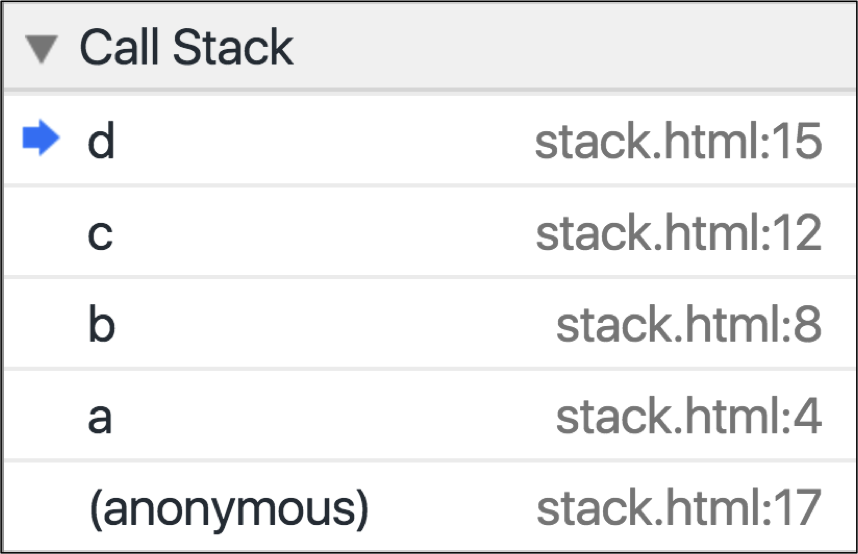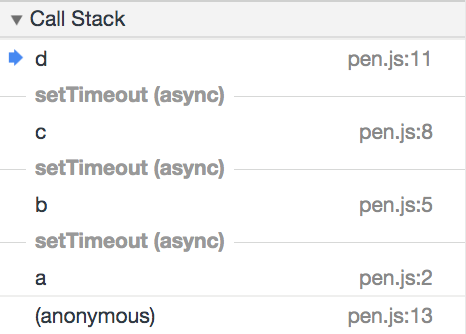# Call Stack `page`

Learn how to trace how functions are called.

## The problem

What is the name of the function that calls the `end` function below?

``````<script src="//bitovi.github.io/academy/static/scripts/debugging/variables.js"></script>
<script type="module">
function end() {
console.log("What is the name of the function that calls end?");
debugger;
}
start();
</script>
``````

## What you need to know

In the following code, `a()` calls `b()`, `b()` calls `c()`, and `c()` calls `d()`:

``````function a(){
var varA = "A";
b();
}
function b(){
var varB = "B";
c();
}
function c(){
var varC = "C";
d();
}
function d(){
var varD = "D";
}
a();
``````

By adding a breakpoint in `d()`, you will see the following in the Call Stack:Chrome can also track asynchrounous function calls. In the following code, `setTimeout` is used to call the next function:

``````function a(){
setTimeout(b,10);
}
function b(){
setTimeout(c,10);
}
function c(){
setTimeout(d,10);
}
function d(){
console.log("d");
}
a();
``````

By adding a breakpoint in `d()`, you will see the following in the Call Stack:## The solution

The answer is `e`.